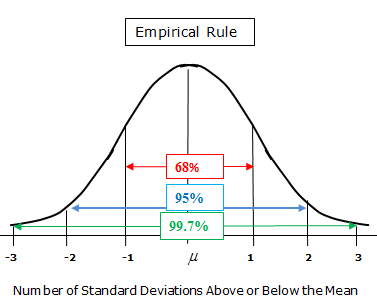MathsGee is Zero-Rated (You do not need data to access) on: Telkom |Dimension Data | Rain | MWEB

2 like 0 dislike
1,473 views
What is the empirical rule for normal distribution?
| 1,473 views

3 like 0 dislike

Statistical rule that states the normal distribution which falls within the three standard deviations 68% falls within the first standard deviation (µ ± σ), 95% within the first two standard deviations (µ ± 2σ), and 99.7% within the first three standard deviations (µ ± 3σ).by Wooden (238 points)
2 like 0 dislike
The empirical rule states that for a normal distribution, nearly all of the data will fall within three standard deviations of the mean. The empirical rule can be broken down into three parts: 68% of data falls within the first standard deviation from the mean. 95% fall within two standard deviations.
by Wooden (1,280 points)
2 like 0 dislike
In normal distribution the empirical rule states that the data falls into three standard deviations of the mean with the first being 68%, second deviation being 95 and the last being 99,7 %
by Wooden (168 points)
1 like 0 dislike
It's a rule that is also known as a 68%, 95% and 99.7% rule. This is because it generally gives the percentage when we distribute from the the mean on a standard distributions that are broken down into three parts on a symmetrical chart.
by Wooden (610 points)
0 like 0 dislike
They call it the 68-95-99.7 rule. It applies for all normally distributed data whereby a bi-directional deviance from the mean of one standard deviation accounts for 68%of the probability. Increasing the standard deviation by 1 unit twice achieves 95% and 99.7% respectively.
by Bronze Status (8,634 points)
1 0
it is the 68,95,99.7 percent rule, where the data of one standard deviation from the mean is 68% ,95,99.7 respectively

0 like 0 dislike
0 like 0 dislike
0 like 0 dislike
1 like 0 dislike